## Wednesday, December 24, 2008

### 2009

2009 = 72 x 41.

2009 has a representation as a sum of two squares: 2009 = 282 + 352.

2009 is an apocalyptic power because 22009 contains the decimal digit string 666.

2009 has been designated the International Year of Astronomy, coinciding with the 400th anniversary of the first recorded astronomical observations with a telescope (Galileo Galilei) and the publication of Astronomia Nova (Johannes Kepler). The United Nations has also designated 2009 the International Year of Natural Fibres.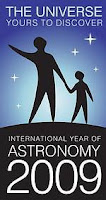In the United States,
2009 is the Year of Science, presented by the Coalition on the Public Understanding of Science (COPUS).

Source:
Number Gossip

## Tuesday, December 23, 2008

### 17

17 is a prime number. It is the third Fermat prime: 17 = 22^2 + 1.

17 has a representation as a sum of two squares: 17 = 12 + 42.

17 is the smallest number with four representations as a sum of three primes: 17 = 2 + 2 + 13 = 3 + 3 + 11 = 3 + 7 + 7 = 5 + 5 + 7.

17 and 19 form a twin prime pair.

17 is the hypotenuse of a primitive Pythagorean triple: 172 = 82 + 152.

17 is the first sum of two distinct fourth powers: 17 = 14 + 24.

There are
17 ways to express 17 as the sum of one or more primes; 17 is the only integer that is equal to the number of prime partitions of itself.Carl Friedrich Gauss proved at the age of 18 that one can construct a regular polygon with a prime number of sides with the use of only straight edge and compasses only if the number of sides is of the form 2
2^n. Hence, one can construct a regular 17-gon with rule and compasses only.

Source: Number Gossip

## Monday, December 22, 2008

### 1717

1717 = 17 x 101.

1717 has two representations as a sum of two squares: 1717 = 62 + 412 = 142 + 392.

1717 is the hypotenuse of two primitive Pythagorean triples: 17172 = 4922 + 16452 = 10922 + 13252.

1717 is a divisor of 1008 - 1.French mathematician and encylopaedist Jean le Rond d'Alembert was born on Nov. 16,
1717.Photo credit: MathDL Portrait Gallery

Source: Number Gossip

## Friday, December 19, 2008

### 1233

1233 = 32 x 137.

1233 has a representation as a sum of two squares: 1233 = 122 + 332. 1233 is the smallest 2n-digit number that is the sum of the squares of its n-digit halves.

1233 is a Happy-Go-Lucky number (both Happy and Lucky).Southern Pacific steam locomotive
1233 was built in 1918 by the Baldwin Locomotive Works in Pennsylvania.

## Thursday, December 18, 2008

### 6141

6141 = 3 x 23 x 89. It is the product of three distinct primes whose binary expansion is palindromic: 1011111111101.

6141 is a Kaprekar constant in base 2.

6141 is the sum of 11 distinct powers of 2.

6141 is a divisor of 222 - 1.6141 Durda is a Mars-crossing asteroid discovered in 1992.

Source: JPL

## Wednesday, December 17, 2008

### 1223

1223 is a prime number. It is a Sophie Germain prime because 2 x 1223 + 1 = 2447, which is also prime.

1223 is a number that cannot be written as a sum of three squares.

1223 is a prime p such that p + 3 is a semiprime: 1223 + 3 = 1226 = 2 x 613.

1223 is a number n such that n and n + 8 are both primes: 1223 and 1231.

1223 is the smallest number with complexity 24. The complexity of an integer is the least number of 1s needed to represent it using only additions, multiplications, and parantheses.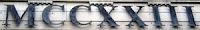1223 Neckar is an asteroid discovered in 1931.

## Tuesday, December 16, 2008

### 866

866 = 2 x 433.

866 is the smallest number n such that the sum of the proper divisors of n2, excluding 1, is a square.

866 has a representation as a sum of two squares: 866 = 52 + 292.

866 is the difference of two positive cubes.866 is one of the telephone area codes for toll-free calls.

Source: Number Gossip

## Monday, December 15, 2008

### 738

738 = 2 x 32 x 41.

738 = 6 + 66 + 666.

738 has a representation as a sum of two squares: 738 = 32 + 272.

738 is a 13-gonal number.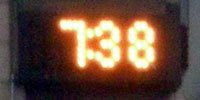The SVI 738 - Xpress was a home computer manufactured by Spectravideo in 1986.

## Friday, December 12, 2008

### 495

495 = 32 x 5 x 11.

495 is the Kaprekar constant for three-digit numbers. Arrange the digits of any three-digit number whose digits are not all the same in descending and ascending order, compute the difference, then repeat the process. For example: 311 - 113 = 198; 981 - 189 = 792; 972 - 279 = 693; 963 - 369 = 594; 954 - 459 = 495.

495 is the ninth pentatope number.

495 is a number that cannot be written as a sum of three squares.Interstate 495 (Capital Beltway) is a highway that surrounds Washington, D.C.

## Thursday, December 11, 2008

### 443

443 is a prime number. It is the 24th Sophie Germain prime: 443 x 2 + 1 = 887, which is also prime.

443 is the first three-digit non-palindromic prime number whose binary equivalent (110111011) is a palindromic prime in base 10.

The sum of the digits of 443 is a prime: 4 + 4 + 3 = 11. Hence, 443 is an additive prime

443 is the smallest prime containing exactly two 4s.Telephone area code 443 includes Baltimore, Md.

## Wednesday, December 10, 2008

### 300

300 = 22 x 3 x 52.

300 is a triangular number, the sum of all positive integers up to 24: 1 + 2 + 3 + . . . + 22 + 23 + 24 = 300.

300 is the sum of twin primes: 149 + 151 = 300.

300 is a number n such that n2 (90000) contains exactly two different digits.In bowling,
300 is the largest possible score.

Source:
Wolfram MathWorld

## Tuesday, December 9, 2008

### 255

255 = 3 x 5 x 17.

255 = 11111111 in base 2.

255 is the largest value representable as an 8-bit binary number. Eight bits, otherwise known as a byte, is a very common size for storing "small" values in computer memories. Consequently, 255 appears as a bound or an otherwise magic number in many computer-related tasks. For example, 255 is the maximum amount of red, green, or blue that goes into an RGB color, as well as the maximum content of any of the four octets of an IP address.

255 is the least odd integer greater than 3 that is not the sum of two distinct powers of two and a prime.

255 is a number that cannot be written as a sum of three squares.

255 is 3333 in base 4.

255 is a Mersenne number: 255 = 28 - 1.Source:
Number Gossip

## Monday, December 8, 2008

### 249

249 = 3 x 83.

The sum of the digits of 249 equals five times the number of digits: 2 + 4 + 9 = 15 = 3 x 5.

249 is a number not divisible by any of its digits.

249 is a Blum number. A natural number n is a Blum integer if n = pq is a semiprime (the product of two prime numbers) for which p and q are distinct prime numbers congruent to 3 mod 4. That is, p and q must be of the form 4t + 3, for some integer t.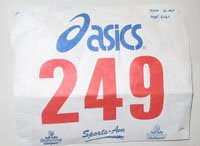249 Ilse is an asteroid with an unusually slow rotation period, discovered in 1885.

## Friday, December 5, 2008

### 146

146 = 2 x 73.

The absolute difference between any two digits of
146 is a prime number (2, 3, 5).

146 is 222 in base 8.

146 is an octahedral number, which has the form (2n3 + n)/3.

146 is an untouchable number: No integer's proper divisors add up to 146.

146 has a representation as a sum of two squares: 146 = 52 + 112.The
Grand Union Canal Race is a 146-mile ultramarathon from Birmingham to London.

## Thursday, December 4, 2008

### 91

91 = 7 x 13.

91 is the first non-trivial odd composite number. Every smaller composite is either even, a familiar square, ends in 5, has a digit sum that is a multiple of 3, or is obviously divisible by 11.

91 is the sum of the squares of the first six integers: 12 + 22 + 32 + 42 + 52 + 62 = 91. It is the 13th triangular number.

91 is the sum of the first 13 positive integers: 1 + 2 + 3 + . . . + 11 + 12 + 13 = 91.

91 has a unique representation as a sum of three squares: 91 = 12 + 32 + 92.

91 is 111 in base 9.91 cents is the total amount of money one has, given one each of a penny, nickel, dime, quarter, and half dollar.Source: Number Gossip

## Wednesday, December 3, 2008

### 51

51 = 3 x 17.

51 is the smallest number that can be written with all the digits from 1 to 5 (without repetition) as a sum of prime numbers: 2 + 3 + 5 + 41 = 51. Note that the highest digit (5) and the lowest digit (1) are the digits of 51.

51 is a pentagonal number, a number of the form n(3n - 1)/2, and a centered pentagonal number (one of the few numbers to be both).

51 is 110011 in base 2 (binary) and 33 in base 16.

51 is the sixth Motzkin number.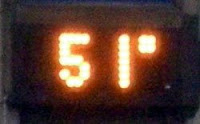Area 51 is a nickname for a secret military base located in the southern portion of Nevada in the western United States.

Source: Prime Curios!

## Tuesday, December 2, 2008

### 47

47 is a safe prime number. A safe prime has the form 2p + 1, where p is also a prime.

There are 47 occurrences of 47 in the first thousand prime numbers.

43 + 73 = 407.

47 is the smallest number n for which 666n has a digit sum of 666.The atomic number of silver is 47.

## Monday, December 1, 2008

### 711

711 = 32 x 79.

711 is the concatenation of the fourth and fifth primes.

711 is a number that cannot be written as a sum of three squares.

711 is a divisor of 802 - 1.

711 is the sum of ten positive fifth powers: 711 = 35 + 35 + 25 + 25 + 25 + 25 + 25 + 25 + 25 + 15.

711 is the phone number of the Telecommunications Relay Service, commonly used by the deaf and hard-of-hearing.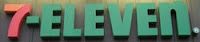The logo for a chain of convenience stores.

#711 was a comics superhero created by George Brenner and published by Quality Comics from 1941 to 1943.

In the 1950 movie 711 Ocean Drive, a telephone repairman in Los Angeles uses his knowledge of electronics to help a bookie set up a betting operation.

Source: WolframAlpha